# Machine Learning 101: Outliers introductionBuilding a Machine Learning might become easier by the day, but there’s a rule of thumb: garbage input equals garbage predictions. Outliers are observation that significantly differ from other observations. Having outliers in your data will hinder you models, let’s discover what they are, how to detect them and remove them.

## A first encounter with Outliers

In statistics, an outlier is a data point that differs significantly from other observations. An outlier may be due to variability in the measurement or it may indicate experimental error; the latter are sometimes excluded from the data set. An outlier can cause serious problems in statistical analyses.

Wikipedia, the free encyclopedia (page)

As mentioned, an outlier is somewhat of an anomaly in data. Most of real-world data that you will encounter during your journey in data science and machine learning will include some outliers. Many Machine Learning models struggle with outliers, models that are not sensitive to outliers are called robust models.

It is therefore essential for any data scientist to know what an outlier looks like, how to detect and remove outliers, and most importantly why this should be done. In the following notebook I will show you a common method used to detect (and remove) outliers. This method is based on Quartiles and the Interquartile Range (IQR), it is integrated in most plotting libraries and it is known as “Tukey’s Fences“.

## Outliers in fish

The following notebook uses the Fish market dataset available here, it is free and released under the GPL2 license. This dataset includes a number of species of fish and for each fish some measurements such as weight and height.

### (Basic) Explanatory Data Analysis

Every good ML algorithm should start with an in-depth Explanatory Data Analysis (EDA). In the EDA you should always try to explore the data as much as possible, through exploration it is possible to infer basic features of the data, from those basic inferences you can start developing a basic intuition. From there you can start formulating hypotheses and implement the algorithm you see fit.

As the purpose of this notebook is to illustrate what outliers are it will only cover this topic.

Firstly, let's import basic utilities:

In :
%matplotlib inline
import pandas as pd
import numpy as np
import matplotlib.pyplot as plt
import seaborn as sns

# Some font improvements
plt.rc('font', size=14)
plt.rc('axes', titlesize=14)
plt.rc('axes', labelsize=14)


Let's now read the csv file (keep in mind it should be in the same folder as the notebook!):

In :
df = pd.read_csv('Fish.csv')


Let's take a look at the first rows of the dataset. It is important to do this in order to get a basic understanding.

In :
df.head()

Out:
Species Weight Length1 Length2 Length3 Height Width
0 Bream 242.0 23.2 25.4 30.0 11.5200 4.0200
1 Bream 290.0 24.0 26.3 31.2 12.4800 4.3056
2 Bream 340.0 23.9 26.5 31.1 12.3778 4.6961
3 Bream 363.0 26.3 29.0 33.5 12.7300 4.4555
4 Bream 430.0 26.5 29.0 34.0 12.4440 5.1340

Now let's take a closer look to the dataset to get important statistical indicators such as the mean and standard deviation

In :
df.describe()

Out:
Weight Length1 Length2 Length3 Height Width
count 159.000000 159.000000 159.000000 159.000000 159.000000 159.000000
mean 398.326415 26.247170 28.415723 31.227044 8.970994 4.417486
std 357.978317 9.996441 10.716328 11.610246 4.286208 1.685804
min 0.000000 7.500000 8.400000 8.800000 1.728400 1.047600
25% 120.000000 19.050000 21.000000 23.150000 5.944800 3.385650
50% 273.000000 25.200000 27.300000 29.400000 7.786000 4.248500
75% 650.000000 32.700000 35.500000 39.650000 12.365900 5.584500
max 1650.000000 59.000000 63.400000 68.000000 18.957000 8.142000

Let's now plot each numerical feature against each other, in order to get a clear distinction use a palette with high contrast (Viridis) and use the species as color.

In :
sns.pairplot(df, hue='Species', palette='viridis')

Out:
<seaborn.axisgrid.PairGrid at 0x1619e2375c8>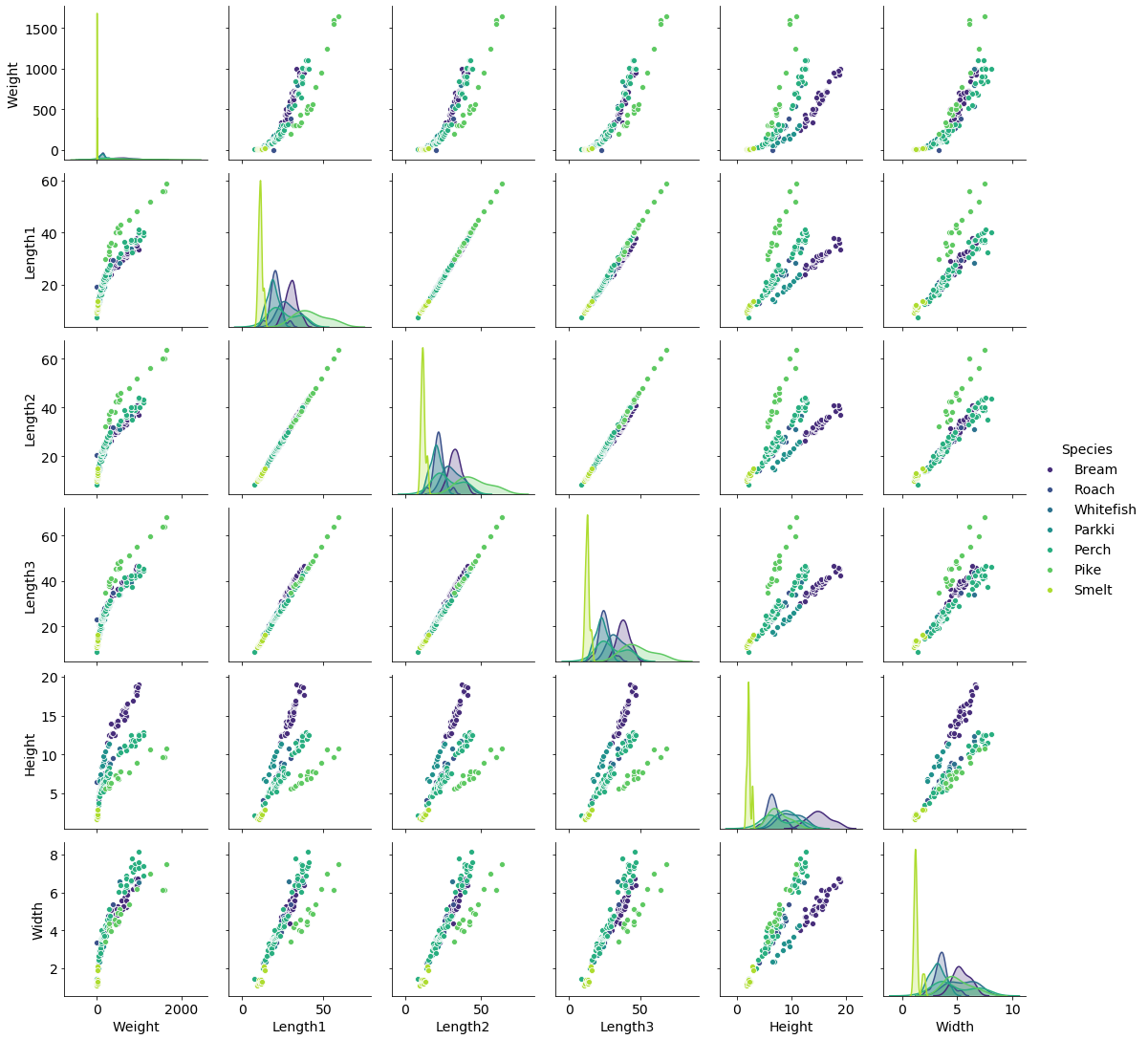Do you notice something strange? Is there some observation that catches your eye?

### Outliers detection using matplotlib/seaborn

Boxplots offer immediate visual feedback to isolate outliers. Outliers are plotted as little diamonds. Can you spot something in the following plots?

In :
fig, axes = plt.subplots(ncols=2, nrows=3, figsize=(15, 18), sharey=False)
sns.boxplot(data=df, y='Weight', x='Species', ax=axes)
sns.boxplot(data=df, y='Width', x='Species', ax=axes)
sns.boxplot(data=df, y='Height', x='Species', ax=axes)
sns.boxplot(data=df, y='Length1', x='Species', ax=axes)
sns.boxplot(data=df, y='Length2', x='Species', ax=axes)
sns.boxplot(data=df, y='Length3', x='Species', ax=axes)

Out:
<matplotlib.axes._subplots.AxesSubplot at 0x1619fa0d248>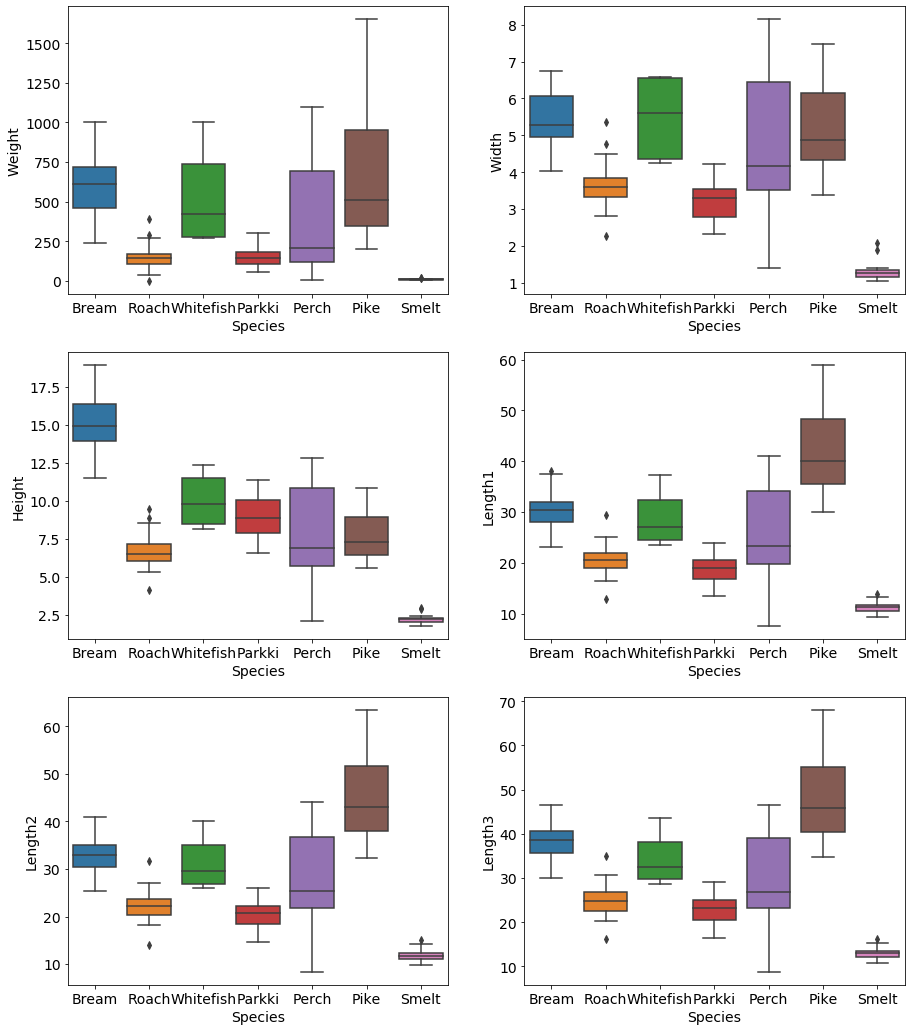### Outliers detection using Tukey's Fences

$$\mathcal{[Q_1 - k(Q_3-Q_1), Q_3 + k(Q_3-Q_1]}$$

Tukey's Fences define a convenient interval for inliers (not outliers). Everything outside the two extremes, should be considered an outlier. $Q_1$ is the first quartile, $Q_3$ is the third quartile, $Q_3-Q_1$ is the Inter-Quartile Range (IQR). $k$ is an arbitrary constant, Tukey proposed 1.5 for outliers, and 3 for "far" outliers. This method is the same used by matplotlib and seaborn with k=1.5 when plotting boxplots.

Let's define the following function to achieve the desired behavior.

In :
def outliers(df, index, k=1.5):
q1 = df[index].quantile(0.25)
q3 = df[index].quantile(0.75)
iqr = q3-q1
outliers = df[(df[index] < q1-k*iqr) | (df[index] > q3+k*iqr)]
return outliers


Let's now take a look at Roach weights.

In :
sns.boxplot(data=df[df['Species'] == 'Roach'], y='Weight', width=0.2)

Out:
<matplotlib.axes._subplots.AxesSubplot at 0x1619fe85188>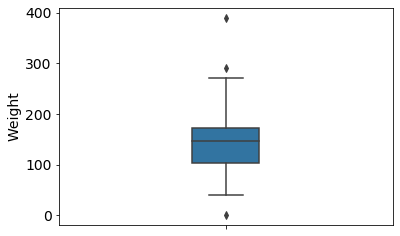In :
outliers(df[df['Species'] == 'Roach'], 'Weight')

Out:
Species Weight Length1 Length2 Length3 Height Width
40 Roach 0.0 19.0 20.5 22.8 6.4752 3.3516
52 Roach 290.0 24.0 26.0 29.2 8.8768 4.4968
54 Roach 390.0 29.5 31.7 35.0 9.4850 5.3550

As you can see there are three outliers among the Roach species when you consider their weight. The first one doesn't even have a weight, so it's an obvious outlier. But what happens if we take a look at the wight of all the species?

In :
outliers(df, 'Weight')

Out:
Species Weight Length1 Length2 Length3 Height Width
142 Pike 1600.0 56.0 60.0 64.0 9.600 6.144
143 Pike 1550.0 56.0 60.0 64.0 9.600 6.144
144 Pike 1650.0 59.0 63.4 68.0 10.812 7.480

The three Roach outliers are now not detected anymore. That is because considering all the species they now fall within the two Tukey's Fences. So are they actually outliers? The answer to this question isn't easy. The fact is that you must always look at the data, if there was some kind of magic algorithm that could delete all outliers without failing it would be at the very base of every ML algorithm, right? You must not overdo when discarding observation, your goal should not be to eliminate the diamonds from each and every boxplot you can think of.

Let's take a look at the other dimensions and their outliers.

In :
fig, axes = plt.subplots(ncols=2, figsize=(15, 5), sharey=False)
sns.boxplot(data=df.drop('Weight', axis=1), ax=axes)
sns.boxplot(data=df, y='Weight', x='Species')

Out:
<matplotlib.axes._subplots.AxesSubplot at 0x161a0426e48>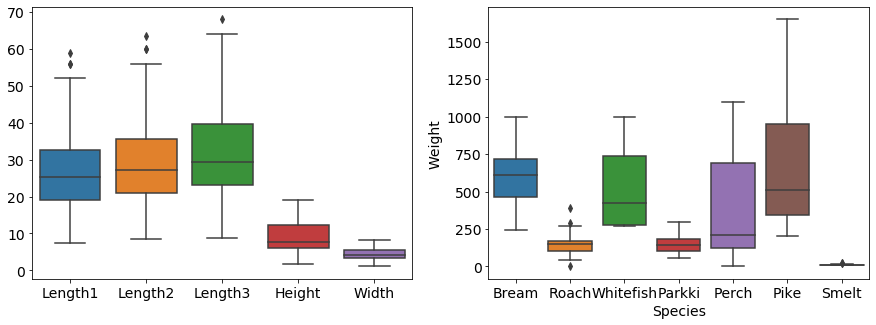Let's take a look once again at the Roach species, this time their Lenght2 feature:

In :
outliers(df[df['Species'] == 'Roach'], 'Length2')

Out:
Species Weight Length1 Length2 Length3 Height Width
35 Roach 40.0 12.9 14.1 16.2 4.1472 2.268
54 Roach 390.0 29.5 31.7 35.0 9.4850 5.355

As you can see the fish that didn't have a weight associated is not here, however the fish with weight equals 390 is. That is an indicator that the observation is indeed an outlier.

### Removing outliers

You can use the following function if you want to remove outliers based on an entire feature. It will return the entire DataFrame without the outliers.

In :
def drop_outliers(df, index, k=1.5):
return df.drop(outliers(df, index, k).index)

In :
df = drop_outliers(df, 'Weight')


If you want to remove outliers based on a class and on a feature (such as weight, species) you will have to do something a bit more complicated:

In :
df = df.drop(outliers(df[df['Species'] == 'Roach'], 'Weight').index)

In :
fig, axes = plt.subplots(ncols=2, figsize=(15, 5), sharey=False)
sns.boxplot(data=df.drop('Weight', axis=1), ax=axes)
sns.boxplot(data=df, y='Weight', x='Species')

Out:
<matplotlib.axes._subplots.AxesSubplot at 0x161a05a0b08>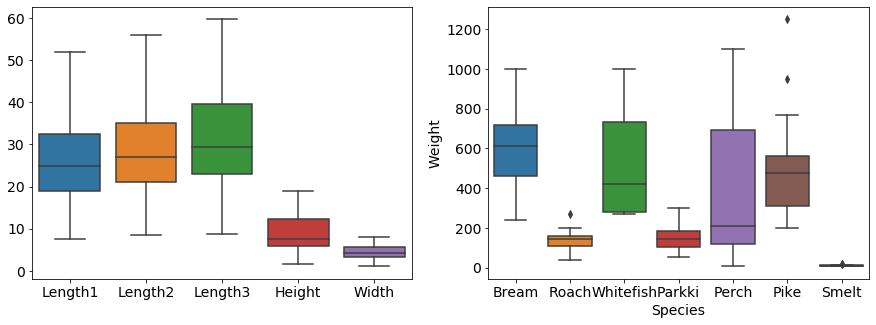### Conclusion

As you can see removing outliers in this way has created other outliers in the Pike species when it comes to weight. Outliers can be harmful to Machine Learning models, but removing them without care may reduce your dataset considerably.

Image courtesy of mark | marksei

The following two tabs change content below.#### mark

The IT guy with a slight look of boredom in his eyes. Freelancer. Current interests: Kubernetes, Tensorflow, shiny new things.#### You may also like...

This site uses Akismet to reduce spam. Learn how your comment data is processed.